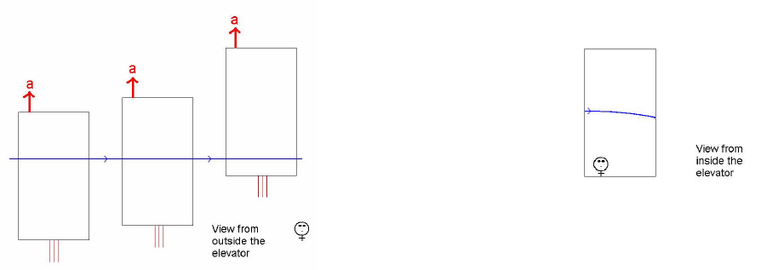Class Notes (1,100,000)
CA (630,000)
UTSG (50,000)
Physics (400)
Lecture 8

# PHY100H1 Lecture 8: L8-9 General Relativity.pdf

Department
Physics
Course Code
PHY100H1
Professor
Vatche Deyirmenjian
Lecture
8

This preview shows half of the first page. to view the full 3 pages of the document.The General Theory of Relativity
Consider the following thought experiments in which air resistance is ignored. Bob is in an
elevator that is stationary at the surface of the Earth. He drops a tennis ball and sees it accelerate
to the floor of the elevator at 10 m/s2. Alice is in an elevator that is far from any stars and is
accelerating upwards at 10 m/s2. She releases a tennis ball. Alice observes it accelerate to the
bottom of the elevator at 10 m/s2 since the floor accelerates upwards at 10 m/s2. This connection
between Alice and Bob’s measurements motivated Einstein to state
The Principle of Equivalence
In a small enough region of space, the results of experiments in a gravitational field are
equivalent to those obtained by an accelerated observer.
The Principle of Equivalence is only valid for a small region of space. How do we describe what
happens in a large region of space? Events such as the collision between a ball and the floor of
an elevator occur at a particular location and time. Space-time is a four-dimensional coordinate
system for identifying events. In Special Relativity, space and time measurements depend on the
motion of observers. Combining three space dimensions (x,y,z) and one time dimension t into a
single system (x,y,z,t) allows for different observers to compare their measurements consistently.
For example, there is an expression involving x, y, z, and t called the space-time distance or
separation that is the same for all observers.
One way of quantifying Bob’s observation is to say that there is a Newtonian gravitational force
or field responsible for the motion of the ball. However, Galileo determined that in the absence
of air resistance all objects, independent of their mass, accelerate at the same rate to the ground.
This is now a well tested fact. Furthermore, there is no gravitational field in Alice’s elevator, but
she sees the ball fall to the floor. This shows that the mass of a falling object and the Newtonian
idea of a gravitational force are not necessary to explain gravity. If the right space-time
coordinate system could be developed, then gravitational effects could be understood in a new
manner. Einstein did this in his General Theory of Relativity, which he finalized in 1915.
In General Relativity (GR), objects and light move along geodesics in space-time. A geodesic is
the path of shortest distance between two events or points in space-time. The geometry or
curvature of space-time is determined by the mass of objects in the universe. The geometry of
space-time may be flat or curved like that of the surface of a table or basketball. Here are some
of the consequences of GR.
Bending of Light in a Gravitational Field